#Function Repository Resource:

# TrimmedLinearFit

Contributed by: Jon McLoone
 ResourceFunction["TrimmedLinearFit"][data,basis,vars] finds a least-squares linear fit of basis to data when 5% of the outliers are discarded. ResourceFunction["TrimmedLinearFit"][data,basis,vars, n] finds a least-squares linear fit of basis to data when n outliers are discarded. ResourceFunction["TrimmedLinearFit"][data,basis,vars, Scaled[n]] finds a least-squares linear fit of basis to data when a proportion n of outliers are discarded.

## Details and Options

If you discard large proportions of the data, the function may not discard the optimal choice of outliers.

## Examples

### Basic Examples

Find a fit ignoring a single outlier:

 In:=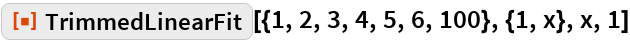Out=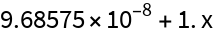Fit would be unduly influenced by the large outlier:

 In:=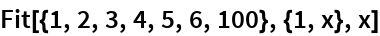Out=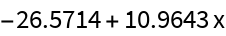In this case, TrimmedLinearFit is broadly equivalent to:

 In:=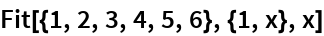Out=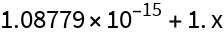### Scope

The amount of trimming can be specified as a proportion of the data, for example, discarding 30% of the data:

 In:=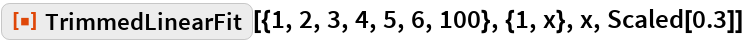Out=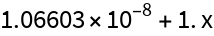Data and models can be multidimensional:

 In:=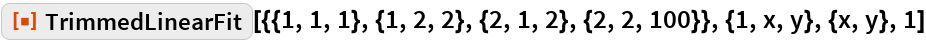Out=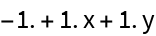## Requirements

Wolfram Language 11.3 (March 2018) or above